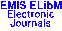PUBLICATIONS DE L'INSTITUT MATHÉMATIQUE (BEOGRAD) (N.S.) Vol. 70(84), pp. 37--41 (2001)

Previous Article

Next Article

Contents of this Issue

Other Issues

ELibM Journals

ELibM Home

EMIS Home

## SPECTRAL RADIUS AND SPECTRUM OF THE COMPRESSION OF A SLANT TOEPLITZ OPERATOR}

### Taddesse Zegeye and S.C. Arora

Department of Mathematics, Bahir Dar University P.O Box 79, Bahir Dar, Ethiopia and Department of Mathematics, University of Delhi, Delhi 110007, India

Abstract: A slant Toeplitz operator $A_\varphi$ with symbol $\varphi$ in $L^\infty(T)$, where $T$ is the unit circle on the complex plane, is an operator whose representing matrix $M=(a_{i j})$ is given by $a_{ij}=\<\varphi, z^{2i-j}\>$, where $\<\cdot,\cdot\>$ is the usual inner product in $L^2(T)$. The operator $B_\varphi$ denotes the compression of $A_\varphi$ to $H^2(T)$(Hardy space). In this paper, we prove that the spectral radius of $B_\varphi$ is greater than the spectral radius of $A_\varphi$, and if $\varphi$ and $\varphi^{-1}$ are in $H^\infty$, then the spectrum of $B_\varphi$ contains a closed disc and the interior of this disc consists of eigenvalues with infinite multiplicity.

Keywords: Toeplitz operator; slant Toeplitz operator; compression; spectrum

Classification (MSC2000): 47B35; 47A10

Full text of the article: